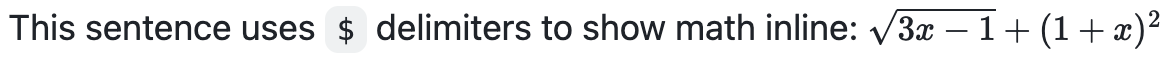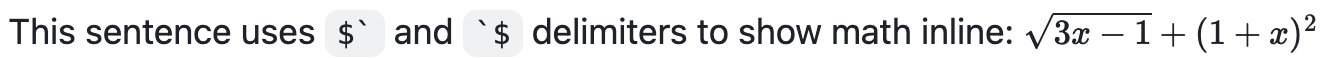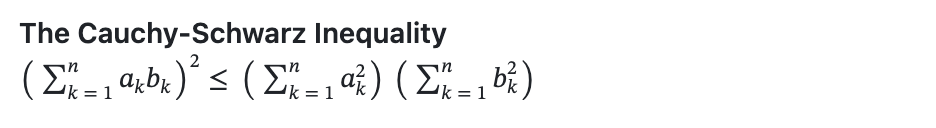설명서에 자주 업데이트를 게시하며 이 페이지의 번역이 계속 진행 중일 수 있습니다. 최신 정보는 영어 설명서를 참조하세요.

# 수학 식 작성

## 이 문서의 내용

Markdown을 사용하여 GitHub에 수학 식을 표시합니다.

To enable clear communication of mathematical expressions, GitHub supports LaTeX formatted math within Markdown. For more information, see LaTeX/Mathematics in Wikibooks.

GitHub's math rendering capability uses MathJax; an open source, JavaScript-based display engine. MathJax supports a wide range of LaTeX macros, and several useful accessibility extensions. For more information, see the MathJax documentation and the MathJax Accessibility Extensions Documentation.

Mathematical expressions rendering is available in GitHub Issues, GitHub Discussions, pull requests, wikis, and Markdown files.

## Writing inline expressions

There are two options for delimiting a math expression inline with your text. You can either surround the expression with dollar symbols ($), or start the expression with $ and end it with $. The latter syntax is useful when the expression you are writing contains characters that overlap with markdown syntax. For more information, see "Basic writing and formatting syntax." This sentence uses $ delimiters to show math inline:  $\sqrt{3x-1}+(1+x)^2$This sentence uses $\ and \$ delimiters to show math inline:  $\sqrt{3x-1}+(1+x)^2$## Writing expressions as blocks

To add a math expression as a block, start a new line and delimit the expression with two dollar symbols $$. **The Cauchy-Schwarz Inequality**$$\left( \sum_{k=1}^n a_k b_k \right)^2 \leq \left( \sum_{k=1}^n a_k^2 \right) \left( \sum_{k=1}^n b_k^2 \right)$$Alternatively, you can use the math code block syntax to display a math expression as a block. With this syntax, you don't need to use $$ delimiters. The following will render the same as above:

**The Cauchy-Schwarz Inequality**

math
\left( \sum_{k=1}^n a_k b_k \right)^2 \leq \left( \sum_{k=1}^n a_k^2 \right) \left( \sum_{k=1}^n b_k^2 \right)



## Writing dollar signs in line with and within mathematical expressions

To display a dollar sign as a character in the same line as a mathematical expression, you need to escape the non-delimiter $ to ensure the line renders correctly. • Within a math expression, add a \ symbol before the explicit $.

This expression uses \$ to display a dollar sign:$\sqrt{\$4}$• Outside a math expression, but on the same line, use span tags around the explicit $. To split <span>$</span>100 in half, we calculate $100/2$
`